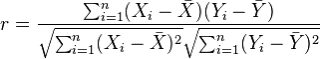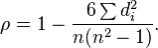# Correlation

Correlation is one of the most common statistics. Using one single value, it describes the “degree of relationship” between two variables. Correlation ranges from -1 to +1. Negative values of correlation indicate that as one variable increases the other variable decreases. Positive values of correlation indicate that as one variable increase the other variable increases as well. There are three options to calculate correlation in R, and we will introduce two of them below.

For a nice synopsis of correlation, see Pearson Product-Moment Correlation - When you should run this test, the range of values the coefficient can take and how to measure strength of association.

## Pearson Correlation

The most commonly used type of correlation is Pearson correlation, named after Karl Pearson, introduced this statistic around the turn of the 20th century. Pearson’s r measures the linear relationship between two variables, say X and Y. A correlation of 1 indicates the data points perfectly lie on a line for which Y increases as X increases. A value of -1 also implies the data points lie on a line; however, Y decreases as X increases. The formula for r is(in the same way that we distinguish between Ȳ and µ, similarly we distinguish r from ρ)

The Pearson correlation has two assumptions:

1. The two variables are normally distributed. We can test this assumption using

2. A statistical test (Shapiro-Wilk)

3. A histogram

4. A QQ plot

5. The relationship between the two variables is linear. If this relationship is found to be curved, etc. we need to use another correlation test. We can test this assumption by examining the scatterplot between the two variables.

To calculate Pearson correlation, we can use the cor() function. The default method for cor() is the Pearson correlation. Getting a correlation is generally only half the story, and you may want to know if the relationship is statistically significantly different from 0.

• H0: There is no correlation between the two variables: ρ = 0
• Ha: There is a nonzero correlation between the two variables: ρ ≠ 0

## Spearman’s rank correlation

Spearman’s rank correlation is a nonparametric measure of the correlation that uses the rank of observations in its calculation, rather than the original numeric values. It measures the monotonic relationship between two variables X and Y.

That is, if Y tends to increase as X increases, the Spearman correlation coefficient is positive. If Y tends to decrease as X increases, the Spearman correlation coefficient is negative. A value of zero indicates that there is no tendency for Y to either increase or decrease when X increases. The Spearman correlation measurement makes no assumptions about the distribution of the data.

The formula for Spearman’s correlation ρs iswhere di is the difference in the ranked observations from each group, (xi – yi), and n is the sample size. No need to memorize this formula!